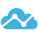จำนวนเข้าชม 3021
3021
This indicator shows the support and resistance levels of four periods. Now you can customize it entirely, defining the periods and what you can show, or not.

When a weaker support/resistance is broken you now what is the next support/resistance .

The average of support and resistance can be used as an entry point or as a indicator of bear/bull market (when the close price is below the average the market is bear, and when the close price is above the average the market is bull).

To add to your chart, add this to favorites and in your chart go to indicators, then to favorites, and it will appear on the right side.

Please, give-me your feedback! And a Tip if you will use it.
study("Dynamic S&R",overlay=true)

// Author: samuelhei
// Tips: 1NR9tyn8tAn6nuQzosaZ2SkfT38PLxN5mf
// Feel free to use this script as you want!

a = input(400,"A (bars back)")
ha = input(true,"Habilitate A",type=bool)
hb = input(true,"Habilitate B",type=bool)
hc = input(true,"Habilitate C",type=bool)
hd = input(true,"Habilitate D",type=bool)

cb = input(0,"Custom B 0=(A/2)",type=integer)
cc = input(0,"Custom C 0=(B/2)",type=integer)
cd = input(0,"Custom D 0=(C/2)",type=integer)

hac = input(true,"Habilitate Average of support and resistance of D", type=bool)
avm = input(1,"Multiplier of averege line.", type=float)

b = cb ? cb : a/2
c = cc ? cc :b/2
d = cd ? cd :c/2

hga = highest(close,a)
hgb = highest(close,b)
hgc = highest(close,c)
hgd = highest(close,d)

la = lowest(close,a)
lb = lowest(close,b)
lc = lowest(close,c)
ld = lowest(close,d)

pha = plot(ha ? hga : na,"Highest A",gray)
phb = plot(hb ? hgb : na,"Highest B",gray)
phc = plot(hc ? hgc : na,"Highest C",gray)
phd = plot(hd ? hgd : na,"Highest D",gray)

fill(pha,phb,black,70)
fill(phb,phc,black,80)
fill(phc,phd,black,90)

pla = plot(ha ? la : na,"Lowest A",gray)
plb = plot(hb ? lb : na,"Lowest B",gray)
plc = plot(hc ? lc : na,"Lowest C",gray)
pld = plot(hd ? ld : na,"Lowest D",gray)

fill(pla,plb,black,70)
fill(plb,plc,black,80)
fill(plc,pld,black,90)

av = ((hgd + ld) / 2)*avm

plot(hac ? av : na,"Average",black)

## ความคิดเห็นThis is actually extremely useful, thanks
ตอบกลับthis looks really interesting and very close to Dynamic Accumulation/ Distribution on Ninjatrader, which I have been searching for for Tradingview. I notice that the lines move as I zoom in and out of the chart. If they are formed on the price action of the last set number of bars, shouldnt they be somewhat locked in place like the Ninjatrader version here: https://www.youtube.com/watch?v=jsf2szUB5bU ?
ตอบกลับ